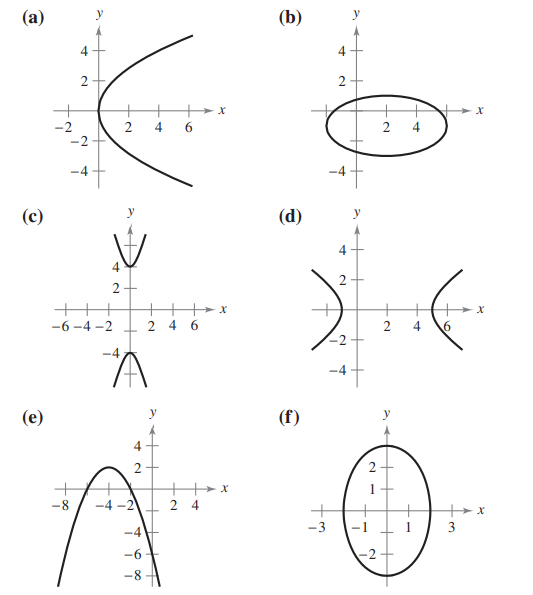×
Get Full Access to Calculus: Early Transcendental Functions - 6 Edition - Chapter 10.1 - Problem 4
Get Full Access to Calculus: Early Transcendental Functions - 6 Edition - Chapter 10.1 - Problem 4

×

# ?In Exercises 1–6, match the equation with its graph. [The graphs are labeled (a), (b), (c), (d), (e), and (f).] $$\ISBN: 9781285774770 141 ## Solution for problem 4 Chapter 10.1 Calculus: Early Transcendental Functions | 6th Edition • Textbook Solutions • 2901 Step-by-step solutions solved by professors and subject experts • Get 24/7 help from StudySoup virtual teaching assistantsCalculus: Early Transcendental Functions | 6th Edition 4 5 1 238 Reviews 30 5 Problem 4 In Exercises 1–6, match the equation with its graph. [The graphs are labeled (a), (b), (c), (d), (e), and (f).]\(\frac{(x-2)^{2}}{16}+\frac{(y+1)^{2}}{4}=1$$

Text Transcription:

(x-2)^\2 / 16 + (y + 1)^2 / 4 = 1

Step-by-Step Solution:

Step 1 of 5) Proof Part (1). If ƒ(c) 6 0, then ƒ(x) 6 0 on some open interval I containing the point c, since ƒ is continuous. Therefore, ƒ is decreasing on I. Since ƒ(c) = 0, the sign of ƒ changes from positive to negative at c so ƒ has a local maximum at c by the First Derivative Test.

Step 2 of 2

## Discover and learn what students are asking

Unlock Textbook Solution